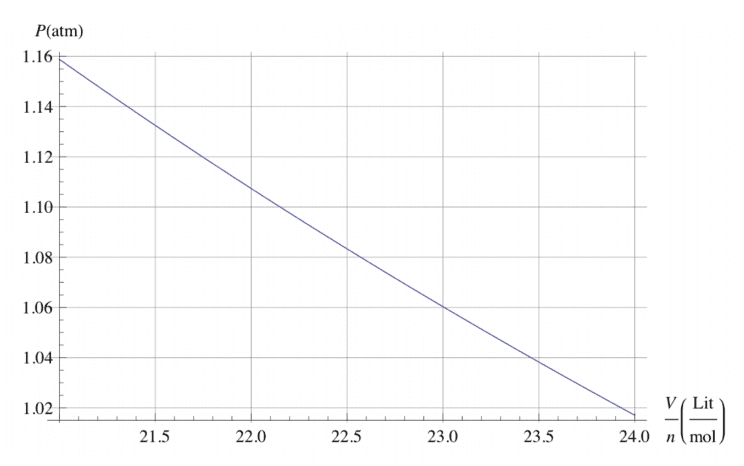# A CAP question related to Van der Waals equation.

• aiyiaiyiai

#### aiyiaiyiai

Homework Statement
The equation of state for some gases can be approximately expressed in the following way:
(P + a*n^2/V^2 )V = nRT
where P is pressure, V is volume, n is the number of moles of a substance, V /n is molar volume, the volume of 1 mole of gas, T is temperature, R ≈ 8.3144621 J/mol·K is the gas constant, a is substance-specific van der Waals constant, in units of kPaL^2/mol^2 , where L means litres.
The following diagram shows the pressure of carbon disulphide gas in terms of volume per mole at temperature of 300 K. What is the van der Waals constant for this gas?
Relevant Equations
Maybe the typical form of van der waals equation?
And this is a MC question, answer is 1300.
Diagram is here.